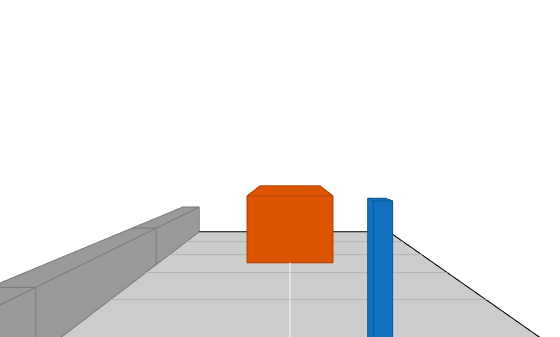# plotBarrierOutline

Display barrier outlines on bird's-eye plot

Since R2021a

## Syntax

``plotBarrierOutline(olPlotter,barrierSegments,positions,yaw,length,width)``
``plotBarrierOutline(___,Name,Value)``

## Description

````plotBarrierOutline(olPlotter,barrierSegments,positions,yaw,length,width)` displays the rectangular outlines of barriers on a bird's-eye plot. Specify the barrier, as well as the position, yaw angle of rotation, length, and width of each barrier segment. The outline plotter `olPlotter`, is associated with a `birdsEyePlot` object, and configures the display of the specified outlines.To remove all outlines associated with the outline plotter `olPlotter`, use the `clearData` function and specify `olPlotter` as the input argument.To display the outlines of the barriers that are in a driving scenario, first use `targetOutlines` with the `'Barriers'` flag as input, to get the dimensions of the barriers. Then, use the `plotBarrierOutline` function to display the outlines of all the barriers in a bird's-eye plot.```

````plotBarrierOutline(___,Name,Value)` specifies options using one or more `Name,Value` pair arguments and the input arguments from the previous syntax.```

## Examples

collapse all

Create a driving scenario. Create a 25 m road segment with a barrier on its left edge. Add a pedestrian that crosses the road at 1 m/s, and a vehicle that drives along the road at 10 m/s.

```scenario = drivingScenario; r = road(scenario,[0 0 0; 25 0 0]); barrier(scenario,r,'RoadEdge','left') p = actor(scenario,'ClassID',4,'Length',0.2,'Width',0.4,'Height',1.7); v = vehicle(scenario,'ClassID',1); smoothTrajectory(p,[15 -3 0; 15 3 0],1) smoothTrajectory(v,[v.RearOverhang 0 0; 25-v.Length+v.RearOverhang 0 0],10)```

Use a chase plot to display the scenario from the perspective of the vehicle.

`chasePlot(v,'Centerline','on')`Create a bird's-eye plot, outline plotter, and lane boundary plotter.

```bep = birdsEyePlot('XLim',[-25 25],'YLim',[-10 10]); olPlotter = outlinePlotter(bep); lbPlotter = laneBoundaryPlotter(bep); legend('off')```Run the simulation loop. Update the plotter with outlines for the targets.

```while advance(scenario) % Obtain the road boundaries and rectangular outlines. rb = roadBoundaries(v); [position,yaw,length,width,originOffset,color] = targetOutlines(v); [bposition,byaw,blength,bwidth,boriginOffset,bcolor,numBarrierSegments] = targetOutlines(v,'Barriers'); % Update the bird's-eye plotters with the road, actors and barriers. plotLaneBoundary(lbPlotter,rb); plotOutline(olPlotter,position,yaw,length,width, ... 'OriginOffset',originOffset,'Color',color); plotBarrierOutline(olPlotter,numBarrierSegments,bposition,byaw,blength,bwidth, ... 'OriginOffset',boriginOffset,'Color',bcolor); % Allow time for plot to update. pause(0.01) end```## Input Arguments

collapse all

Outline plotter, specified as an `OutlinePlotter` object. This object is stored in the `Plotters` property of a `birdsEyePlot` object and configures the display of the specified outlines in the bird's-eye plot. To create this object, use the `outlinePlotter` function.

Number of barrier segments in each barrier, specified as a nonnegative real-valued M-by-1 vector. M is the number of barriers in the scenario.

Positions of all the barrier segments in the detected barriers, specified as an M-by-2 real-valued matrix of (X,Y) positions. M is the number of barrier segments in the detected barriers.

Angles of rotation for barrier outlines, specified as an M-element real-valued vector, where M is the number of barrier segments in the detected barriers.

Lengths of barrier outlines, specified as an M-element real-valued vector, where M is the number of barrier segments in the detected barriers.

Widths of barrier outlines, specified as an M-element real-valued vector, where M is the number of barrier segments in the detected barriers.

### Name-Value Arguments

Specify optional pairs of arguments as `Name1=Value1,...,NameN=ValueN`, where `Name` is the argument name and `Value` is the corresponding value. Name-value arguments must appear after other arguments, but the order of the pairs does not matter.

Before R2021a, use commas to separate each name and value, and enclose `Name` in quotes.

Example: `'Color'`,`[1 0 0]` changes the color of a barrier outline to red.

Rotational centers of the rectangles relative to origin, specified as the comma-separated pair consisting of `'OriginOffset'` and an M-by-2 real-valued matrix. M is the number of barrier segments in the detected barriers. Each row specifies the rotational center of the rectangle representing the barrier segment outline as an (X,Y) displacement from the geometric center of that rectangle.

Outline color, specified as the comma-separated pair consisting of `'Color'` and an M-by-3 matrix of RGB triplets. M is the number of barrier segments in the detected barriers. If you do not specify this argument, the function uses the default colormap for each object.

Example: `'Color'`,```[0 0.5 0.75; 0.8 0.3 0.1]```

## Version History

Introduced in R2021a